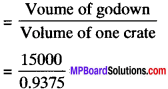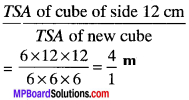# MP Board Class 9th Maths Solutions Chapter 13 Surface Areas and Volumes Ex 13.5

## MP Board Class 9th Maths Solutions Chapter 13 Surface Areas and Volumes Ex 13.5

Assume π = $$\frac{22}{7}$$, unless stated otherwise.

Question 1.
A matchbox measures 4 cm x 2.5 cm. x 1.5 cm. What will be the volume of a packet containing 12 such boxes?
Solution:
Measures of matchbox (cuboid) is 4 cm x 2.5 cm x 1.5 cm
l = 4 cm,
b = 2.5 cm and
h = 1.5 cm
∴ Volume of matchbox = (l x b) x h
= [4 cm x 2.5 cm] x 1.5 cm3
= 4 x $$\frac{25}{10}$$ x $$\frac{25}{10}$$ cm3 = 15 cm3
Volume of 12 boxes =12 x 15 cm3 = 180 cm3Question 2.
A cuboidal water tank is 6 m long, 5 m wide and 4.5 m deep. How many litres of water can it hold? (1 m3 = 1000 l)
Solution:
Here, Length (l) = 6 m
Depth (h) = 4.5 m
Capacity = l x b x h = 6 x 5 x 4.5 m3
= 6 x 5 x $$\frac{45}{10}$$ m3 = 3 x 45
= 135 m3
∴ 1 m3 can hold 1000 l.
∴ 135 m3 can hold (135 x 1000 l = 135000 l) of water.
∴ The required amount of water in the tank = 135000 l.

Question 3.
A cuboidal vessel is 10 m long and 8 m wide. How high must it be made to hold 380 cubic meters of a liquid?
Solution:
Volume of the vessel = l x b x h
380 = 10 x 8 x h
380 = 80 x h
h = $$\frac{380}{80}$$ = 4.75m.

Question 4.
Find the cost of digging a cuboidal pit 8 m long, 6 m broad and 3 m deep at the rate of ₹ 30 per m3.
Solution:
Volume of cuboidal pit = 8 x 6 x 3 = 144 m3
Cost of digging = 144 x 30 = ₹ 4320.

Question 5.
The capacity of a cuboidal tank is 50000 liters of water. Find the breadth of the tank, it its length and depth are respectively 2.5 m and 10 m.
Solution:
Capacity of cuboidal tank = $$\frac{50000}{1000}$$ m3 = 50 m3
Volume of cuboidal tank = l x b x h
50 = 2.5 x 6 x 10
50 = 25b
Breadth of tank, b = $$\frac{50}{25}$$ = 2 m.Question 6.
A village, having a population of4000, requires 150 liters of water per head per day. It has a tank measures 20 m x 15 m x 6m. For how many days will the water of this tank last?
Solution:
Volume of water required for the village per day = $$\frac{4000×150}{1000}$$
Volume of tank = 20 x 15 x 6 = 1800 m3
No. of days = $$\frac{1800}{600}$$ = 3.

Question 7.
A godown measures 60 m x 25 m x 10 m. Find the maxium number of wooden crates each measuring 1.5 m x 1.25 m x 0.5 m that can be stored in the godown.
Solution:
Volume of godown = (60 x 25 x 10) m3 = 15000 m3
Volume of one wooden crate = (1.5 x 1.25 x 0.5) m3 = 0.9375 m3
No. of wooden crates which can be stored in the godown= 16000.

Question 8.
A solid cube of side 12 cm is cut into eight cubes of equal volume. What will be the side of the new cubk? Also, find the ratio between their surface area.
Solution:
Volume of cube of side 12 cm = (12 x 12 x 12) cm3
Let V be volume of new cube.
Volume of cube of side 12 cm = 8 x volume of new cube
12 x 12 x 12 = 8 x V
V = $$\frac{12x12x12}{8}$$ = $$\frac{12x12x12}{2x2x2}$$ = 216 cm3
Let a be the side of new cube
a3 = 216 = 63
a = 6 cm
TSA of cube of side 12 = 6 x (12)2
TSA of new cube = 6 x (6)2Question 9.
Anver 3 m deep and 40 m wide is flowing at the rate of 2 km per hour. How much water will fall into the sea in a minute?
Solution:
b = 40 m,
h = 3 m
V = 2 km/hr
= $$\frac{2×1000}{60}$$ = $$\frac{100}{3}$$ m/min
Volume of water coming out of the river per min = b x h x V
= $$\frac{100}{3}$$ x 3 x 40 = 4000 m3.